﻿

A criterion for a group to be nilpotent

Abstract

Let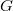$G$ be a group with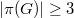$|\pi(G)| \geq 3$. In this paper it is shown that$G$ is nilpotent if and only if for every subgroup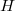$H$ of$G$ with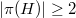$|\pi(H)| \geq 2$ we have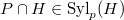$P \cap H \in \mbox{Syl}_{p}(H)$ for each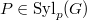$P \in \mbox{Syl}_{p}(G)$ and for every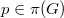$p \in \pi(G)$.

DOI Code: 10.1285/i15900932v20n1p33

Keywords: Nilpotent; Sylow subgroups; Intersections of Sylow subgroups with subgroups

Classification: 20D15; 20D20

Full Text: PDF